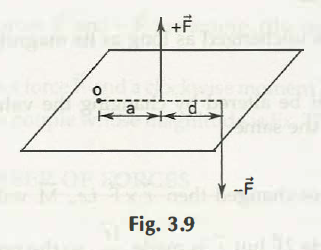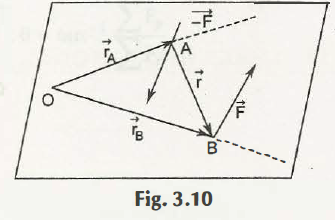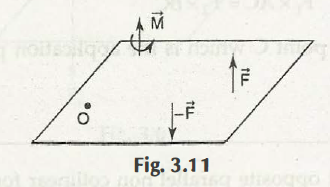When a pair of equal and opposite equivalent non-collinear forces comes in action on a body, then it is called a t-couple force.From the above, we assume that let d be the arm of the pair force. Hence, F and – F forces cannot be united with a single force which results in the sum as zero. This is the reason that when a force acts in the body, then it will have an affinity of rotation. The couple moment M about o has a magnitude

|M|=|Fa –F (a+d)

=| Fa –Fa –Fd

=| -Fd | =Fd .

Thus the result of two forces is equal to the magnitude of the combined moment M which is at a right angle between the distances.

Thus we see in the Fig. 3.9, the couple moment is clockwise and is thus considered as a negative value.

Now the vector algebra is taken into action for the calculation of the couple moment.It is a vital point which has to be remembered that the couple moment M is expressed regarding vector and the reference point is zero. This is applicable for all the moment centers. This is the reason that the couple moment is considered as a free vector which is always perpendicular to the plane of the forces.Links of Previous Main Topic:-

Links of Next Mechanical Engineering Topics:-

### Submit Your Assignment### Customer Reviews

My Homework Help
Rated 5.0 out of 5 based on 510 customer reviews at